## RD Sharma Class 9 Solutions Chapter 10 Congruent Triangles Ex 10.3

These Solutions are part of RD Sharma Class 9 Solutions. Here we have given RD Sharma Class 9 Solutions Chapter 10 Congruent Triangles Ex 10.3

Other Exercises

Question 1.
In the figure, lines l1 and l2 intersect at O, forming angles as shown in the figure. If x = 45, find the values of y, z and n.Solution:
Two lines l1 and l2 intersect each other at O ∠x = 45°
∵ ∠z = ∠x (Vertically opposite angles)
= 45°
But x + y = 180° (Linear pair)
⇒45° + y= 180°
⇒ y= 180°-45°= 135°
But u = y (Vertically opposite angles)
∴ u = 135°
Hence y = 135°, z = 45° and u = 135°

Question 2.
In the figure, three coplanar lines intersect at a point O, forming angles as shown in the figure. Find the values of x, y, z and u.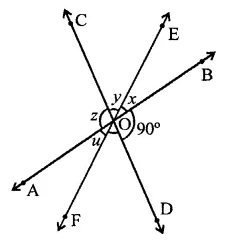Solution:
Three lines AB, CD and EF intersect at O
∠BOD = 90°, ∠DOF = 50°
∵ AB is a line
∴ ∠BOD + ∠DOF + FOA = 180°
⇒ 90° + 50° + u = 180°
⇒ 140° + w = 180°
∴ u= 180°- 140° = 40°
But x = u (Vertically opposite angles)
∴ x = 40°
Similarly, y = 50° and z = 90°
Hence x = 40°, y = 50°, z = 90° and u = 40°

Question 3.
In the figure, find the values of x, y and z.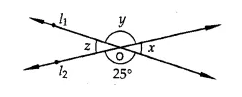Solution:
Two lines l1 and l2 intersect each other at O
∴ Vertically opposite angles are equal,
∴ y = 25° and x = z
Now 25° + x = 180° (Linear pair)
⇒ x= 180°-25°= 155°
∴ z = x = 155°
Hence x = 155°, y = 25°, z = 155°

Question 4.
In the figure, find the value of x.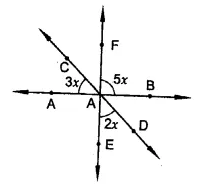Solution:
∵ EF and CD intersect each other at O
∴ Vertically opposite angles are equal,
∴ ∠1 = 2x
AB is a line
3x + ∠1 + 5x = 180° (Angles on the same side of a line)
⇒ 3x + 2x + 5x = 180°
⇒ 10x = 180° ⇒ x = $$\frac { { 180 }^{ \circ } }{ 10 }$$  = 18°
Hence x = 18°

Question 5.
If one of the four angles formed by two intersecting lines is a right angle, then show that each of the four angles is a right angle.
Solution:
Given : Two lines AB and CD intersect each other at O. ∠AOC = 90°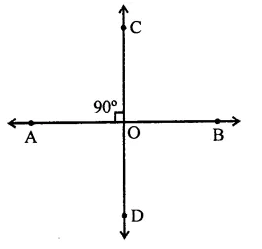To prove: ∠AOD = ∠BOC = ∠BOD = 90°
Proof : ∵ AB and CD intersect each other at O
∴ ∠AOC = ∠BOD and ∠BOC = ∠AOD (Vertically opposite angles)
∴ But ∠AOC = 90°
∴ ∠BOD = 90°
∴ ∠AOC + ∠BOC = 180° (Linear pair)
⇒ 90° + ∠BOC = 180°
∴ ∠BOC = 180° -90° = 90°
∴ ∠AOD = ∠BOC = 90°
∴ ∠AOD = ∠BOC = ∠BOD = 90°

Question 6.
In the figure, rays AB and CD intersect at O.
(i) Determine y when x = 60°
(ii) Determine x when y = 40°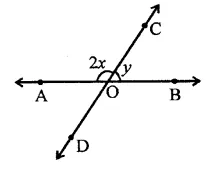Solution:
In the figure,
AB is a line
∴ 2x + y = 180° (Linear pair)
(i) If x = 60°, then
2 x 60° + y = 180°
⇒ 120° +y= 180°
∴ y= 180°- 120° = 60°
(ii) If y = 40°, then
2x + 40° = 180°
⇒ 2x = 180° – 40° = 140°
⇒ x= $$\frac { { 140 }^{ \circ } }{ 2 }$$  =70°
∴ x = 70°

Question 7.
In the figure, lines AB, CD and EF intersect at O. Find the measures of ∠AOC, ∠COF, ∠DOE and ∠BOF.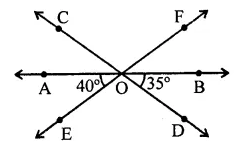Solution:
Three lines AB, CD and EF intersect each other at O
∠AOE = 40° and ∠BOD = 35°
(i) ∠AOC = ∠BOD (Vertically opposite angles)
= 35°
AB is a line
∴ ∠AOE + ∠DOE + ∠BOD = 180°
⇒ 40° + ∠DOE + 35° = 180°
⇒ 75° + ∠DOE = 180°
⇒ ∠DOE = 180°-75° = 105°
But ∠COF = ∠DOE (Vertically opposite angles)
∴ ∠COF = 105°
Similarly, ∠BOF = ∠AOE (Vertically opposite angles)
⇒ ∠BOF = 40°
Hence ∠AOC = 35°, ∠COF = 105°, ∠DOE = 105° and ∠BOF = 40°

Question 8.
AB, CD and EF are three concurrent lines passing through the point O such that OF bisects ∠BOD. If ∠BOF = 35°, find ∠BOC and ∠AOD.
Solution:
AB, CD and EF intersect at O. Such that OF is the bisector of
∠BOD ∠BOF = 35°∵ OF bisects ∠BOD,
∴ ∠DOF = ∠BOF = 35° (Vertically opposite angles)
∴ ∠BOD = 35° + 35° = 70°
But ∠BOC + ∠BOD = 180° (Linear pair)
⇒ ∠BOC + 70° = 180°
⇒ ∠BOC = 180°-70°= 110°
But ∠AOD = ∠BOC (Vertically opposite angles)
= 110°
Hence ∠BOC = 110° and ∠AOD =110°

Question 9.
In the figure, lines AB and CD intersect at O. If ∠AOC + ∠BOE = 70° and ∠BOD = 40°, find ∠BOE and reflex ∠COE.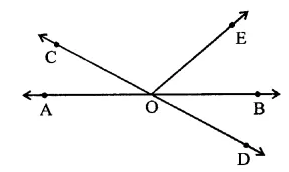Solution:
In the figure, AB and CD intersect each other at O
∠AOC + ∠BOE = 70°
∠BOD = 40°AB is a line
∴ ∠AOC + ∠BOE + ∠COE = 180° (Angles on one side of a line)
⇒ 70° + ∠COE = 180°
⇒ ∠COE = 180°-70°= 110°
and ∠AOC = ∠BOD (Vertically opposite angles)
⇒ ∠AOC = 40°
∴ ∠BOE = 70° – 40° = 30°
and reflex ∠COE = 360° – ∠COE
= 360°- 110° = 250°

Question 10.
Which of the following statements are true (T) and which are false (F)?
(i) Angles forming a linear pair are supplementary.
(ii) If two adjacent angles are equal, then each angle measures 90°.
(iii) Angles forming a linear pair can both be acute angles.
(iv) If angles forming a linear pair are equal, then each of these angles is of measure 90°.
Solution:
(i) True.
(ii) False. It can be possible if they are a linear pair.
(iii) False. In a linear pair, if one is acute, then the other will be obtuse.
(iv) True.

Question 11.
Fill in the blanks so as to make the following statements true:
(i) If one angle of a linear pair is acute, then its other angle will be …….. .
(ii) A ray stands on a line, then the sum of the two adjacent angles so formed is ……… .
(iii) If the sum of two adjacent angles is 180°, then the …… arms of the two angles are opposite rays.
Solution:
(i) If one angle of a linear pair is acute, then its other angle will be obtuse.
(ii) A ray stands on a line, then the sum of the two adjacent angles so formed is 180°.
(iii) If the sum of two adjacent angles is 180°, then the uncommon arms of the two angles are opposite rays.

Question 12.
Prove that the bisectors of a pair of vertically opposite angles are in the same straight line.
Solution:
Given : Lines AB and CD intersect each other at O.
OE and OF are the bisectors of ∠AOC and ∠BOD respectivelyTo prove : OE and OF are in the same line
Proof : ∵ ∠AOC = ∠BOD (Vertically opposite angles)
∵ OE and OF are the bisectors of ∠AOC and ∠BOD
∴ ∠1 = ∠2 and ∠3 = ∠4
⇒ ∠1 = ∠2 = $$\frac { 1 }{ 2 }$$ ∠AOC and
∠3 = ∠4 = $$\frac { 1 }{ 2 }$$ ∠BOD
∴ ∠1 = ∠2 = ∠3 = ∠4
∵ AOB is a line
∴ ∠BOD + ∠AOD = 180° (Linear pair)
⇒ ∠3 + ∠4 + ∠AOD = 180°
⇒ ∠3 + ∠1 + ∠AOD = 180° (∵ ∠1 = ∠4)
∴ EOF is a straight line

Question 13.
If two straight lines intersect each other, prove that the ray opposite to the bisector of one of the angles thus formed bisects the vertically opposite angle.
Solution:
Given : AB and CD intersect each other at O. OE is the bisector of ∠AOD and EO is produced to F.To prove : OF is the bisector of ∠BOC
Proof : ∵ AB and CD intersect each other at O
∴ ∠AOD = ∠BOC (Vertically opposite angles)
∵OE is the bisector of ∠AOD
∴ ∠1 = ∠2
∵ AB and EF intersect each other at O
∴∠1 = ∠4 (Vertically opposite angles) Similarly, CD and EF intersect each other at O
∴ ∠2 = ∠3
But ∠1 = ∠2
∴ ∠3 = ∠4
OF is the bisector of ∠BOC

Hope given RD Sharma Class 9 Solutions Chapter 10 Congruent Triangles Ex 10.3 are helpful to complete your math homework.

If you have any doubts, please comment below. Learn Insta try to provide online math tutoring for you.